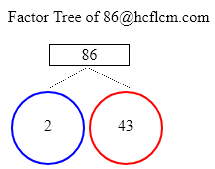# Factor Tree of 86

Created By : Bhagya
Reviewed By : Rajashekhar Valipishetty
Last Updated at : Mar 29,2023

Factor Tree of:### How to find the Factor Tree of 86

Given number is 86
The factor tree of 86 is as follows:

 86 2 4386 = 2 x 43

### Factor Tree Calculations

Here are examples of Factor tree calculations.

### Ways to Find Factors of 86

Here we are providing different methods to find the factors of the number 86. They are splitting numbers and prime factors.

Splitting Numbers

We can divide the number into either of its two factors. In other words, we are looking for the numbers that when multiplied together eqaul 86. Let’s start with 2 x 43 as it results in 86 on multiplying. We will now find the factors or ancestors of split factors, just like in any family tree.

Prime Factors

Let’s take a look at 86 now and we can write as 2 x 43 and place those factors on the tree. similarly to prime numbers obtained in the first step 2, 43 obtained here are also prime numbers and we will end up these branches.

### FAQ on 86 Factor Tree

1. What is factor tree for 86 ?

Answer: Prime Factors of 86 are 2 x 43.

2. What are the Prime Factors of 86 ?

Answer: Prime Factors of 86 are 2 x 43.